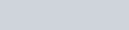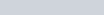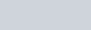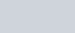• 1
•
•
•
•
•
•
•
1
Share

# CAPILLARY ACTION

Today we are going to talk about the concept of capillarity and further, we will derive an expression for the capillary rise. It is a very important and interesting concept of hydrodynamics.
This concept has many applications in our daily life such as:
• The absorption of ink by the blotting paper is due to capillary action because blotting paper has pores that absorb the ink and slowly ink rises.
• The rising of the oil through the wick of the lamp is also a capillary action.
• The upper portion of the trees gets water and food due to capillarity.
• The absorption of water by the towel after bathing is due to capillarity.
• The rising of water in the handpump is also a capillary action.There are many applications of capillarity in our surroundings if we watch carefully.
So after going further in the article, we have to understand the meaning of capillarity first.

Capillary is taken from the Latin word capila, which means hair-like. So capillary is regarded as a tube that is hair-like thin, and its radius is very small.
And capillarity is the ability of a fluid to flow in narrow spaces in the tube which is hair-like thin, without opposition of any external force like gravity.

But note that, capillarity is able to pull the fluid to some finite height only and then after which gravity start acting. It means capillarity is not totally free from gravity.

## WHY DOES FLUID RISE IN THE CAPILLARY TUBE?

The rise and fall of fluid in the capillary tube depends on the intermolecular forces between the fluid and surrounding solid surface interface. If the diameter of the tube is sufficiently small then the combination of surface tension (which is caused by the cohesion within the fluid) and adhesive forces between the fluid and container wall act to propel the fluid upward.

If the cohesive forces are greater than the adhesive forces then fluid falls in the tube,
This can be seen if we dip a capillary tube in the tub of mercury.
And if the adhesive forces are greater than the cohesive forces then fluid rises in the tube.
Now we are interested in finding the height of fluid at which it rises.
So we are going to derive a formula for the ascent of fluid in a capillary tube.

# CAPILLARY RISE DERIVATION

Let’s take a capillary tube of radius r and insert it into a vessel of fluid which has density ρ and having surface tension T, and pressure outside is P(a) and inside the tube is p0, and θ is the angle of contact, see the following figure.Then the pressure difference in the terms of surface tension is given as:where Tcosθ give rise to the fluid because it is acting upward.
And this pressure difference is also balanced by the fluid column of height h, and it can be written as-Then placing ρgh in the place of pressure difference in the equation of Surface tension. Then we get-Then the height of fluid in the capillary is given as follows:This is the required height gained by the fluid in the capillary tube.